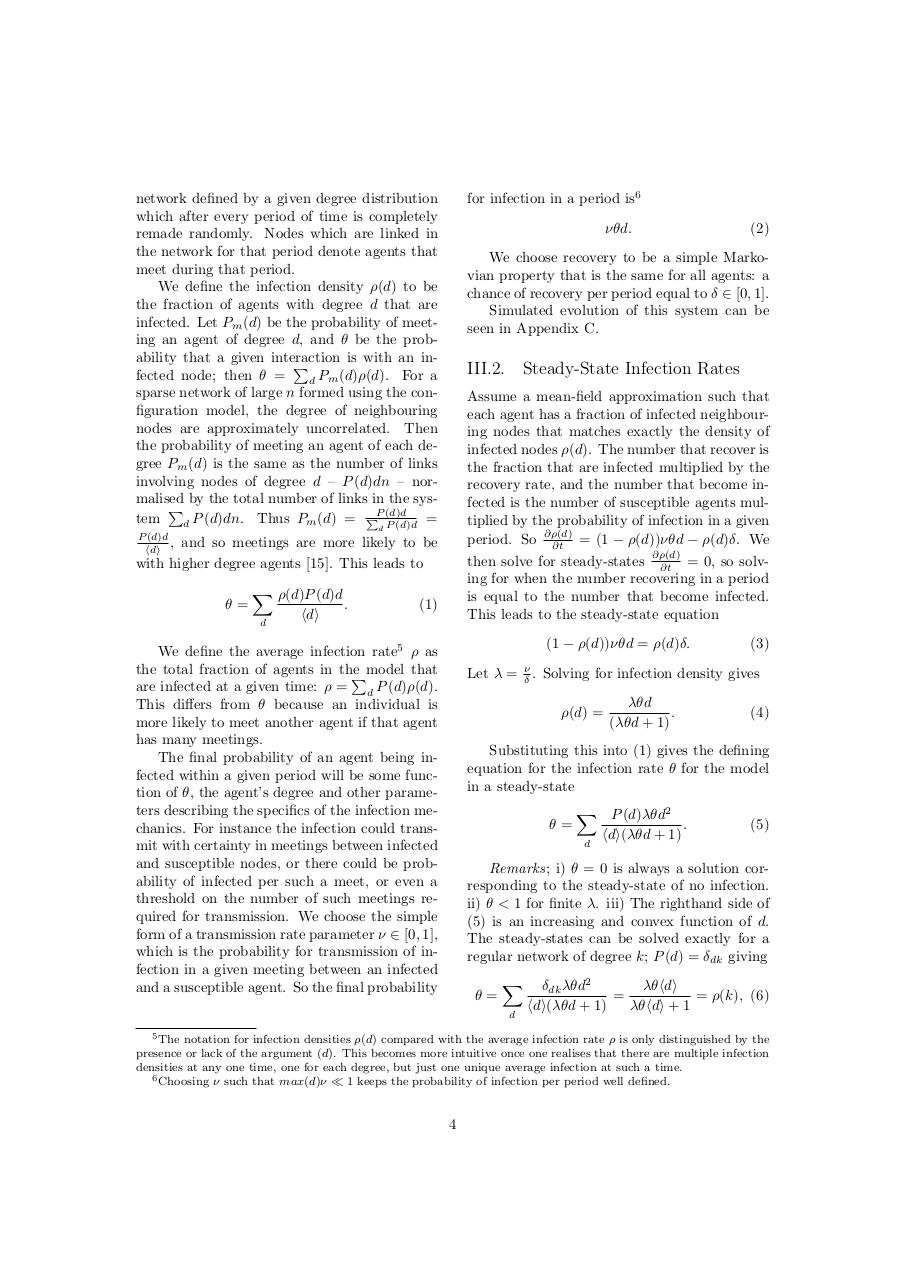# PDF Archive

Easily share your PDF documents with your contacts, on the Web and Social Networks.

## Adaptive Percolation Daniel Burkhardt Cerigo.pdfPage 1 2 3 45624

#### Text preview

for infection in a period is6

network defined by a given degree distribution
which after every period of time is completely
the network for that period denote agents that
meet during that period.
We define the infection density ⇢(d) to be
the fraction of agents with degree d that are
infected. Let Pm (d) be the probability of meeting an agent of degree d, and ✓ be the probability that a given interaction
is with an inP
fected node; then ✓ =
P
(d)⇢(d).
For a
m
d
sparse network of large n formed using the configuration model, the degree of neighbouring
nodes are approximately uncorrelated. Then
the probability of meeting an agent of each degree Pm (d) is the same as the number of links
involving nodes of degree d – P (d)dn – normalised by the total number of links in the sysP
PP (d)d
tem
d P (d)dn. Thus Pm (d) =
P (d)d =

⌫✓d.

We choose recovery to be a simple Markovian property that is the same for all agents: a
chance of recovery per period equal to 2 [0, 1].
Simulated evolution of this system can be
seen in Appendix C.

Assume a mean-field approximation such that
each agent has a fraction of infected neighbouring nodes that matches exactly the density of
infected nodes ⇢(d). The number that recover is
the fraction that are infected multiplied by the
recovery rate, and the number that become infected is the number of susceptible agents multiplied by the probability of infection in a given
period. So @⇢(d)
= (1 ⇢(d))⌫✓d ⇢(d) . We
@t
@t = 0, so solving for when the number recovering in a period
is equal to the number that become infected.

d

P (d)d
hdi ,

and so meetings are more likely to be
with higher degree agents . This leads to
✓=

X ⇢(d)P (d)d
d

hdi

.

(2)

(1)

(1

We define the average infection rate5 ⇢ as
the total fraction of agents in the
Pmodel that
are infected at a given time: ⇢ = d P (d)⇢(d).
This di↵ers from ✓ because an individual is
more likely to meet another agent if that agent
has many meetings.
The final probability of an agent being infected within a given period will be some function of ✓, the agent’s degree and other parameters describing the specifics of the infection mechanics. For instance the infection could transmit with certainty in meetings between infected
and susceptible nodes, or there could be probability of infected per such a meet, or even a
threshold on the number of such meetings required for transmission. We choose the simple
form of a transmission rate parameter ⌫ 2 [0, 1],
which is the probability for transmission of infection in a given meeting between an infected
and a susceptible agent. So the final probability

Let

⇢(d))⌫✓d = ⇢(d) .

(3)

= ⌫ . Solving for infection density gives
⇢(d) =

✓d
.
( ✓d + 1)

(4)

Substituting this into (1) gives the defining
equation for the infection rate ✓ for the model
✓=

X P (d) ✓d2
.
hdi( ✓d + 1)

(5)

d

Remarks; i) ✓ = 0 is always a solution corresponding to the steady-state of no infection.
ii) ✓ &lt; 1 for finite . iii) The righthand side of
(5) is an increasing and convex function of d.
The steady-states can be solved exactly for a
regular network of degree k; P (d) = dk giving
✓=

X
d

5 The

✓d2
✓hdi
=
= ⇢(k), (6)
hdi( ✓d + 1)
✓hdi + 1
dk

notation for infection densities ⇢(d) compared with the average infection rate ⇢ is only distinguished by the
presence or lack of the argument (d). This becomes more intuitive once one realises that there are multiple infection
densities at any one time, one for each degree, but just one unique average infection at such a time.
6 Choosing ⌫ such that max(d)⌫ ⌧ 1 keeps the probability of infection per period well defined.

4